# 1762 Happy Saint Patrick’s Day!

### Today’s ♣ Puzzle:

Here’s a much easier puzzle than yesterday’s for you to enjoy on this Saint Patrick’s Day. The diagonal lines on the corner boxes are only to help define the leaves of the shamrock.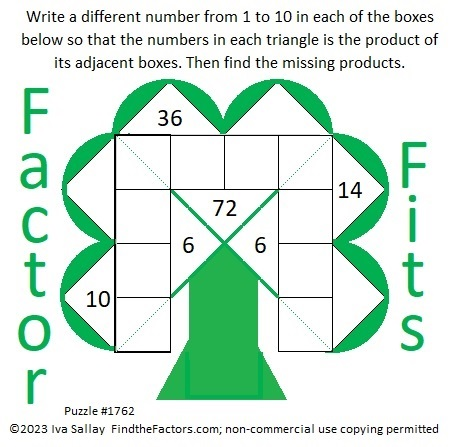### Factors of 1762:

• 1762 is a composite number.
• Prime factorization: 1762 = 2 × 881.
• 1762 has no exponents greater than 1 in its prime factorization, so √1762 cannot be simplified.
• The exponents in the prime factorization are 1 and 1. Adding one to each exponent and multiplying we get (1 + 1)(1 + 1) = 2 × 2 = 4. Therefore 1762 has exactly 4 factors.
• The factors of 1762 are outlined with their factor pair partners in the graphic below.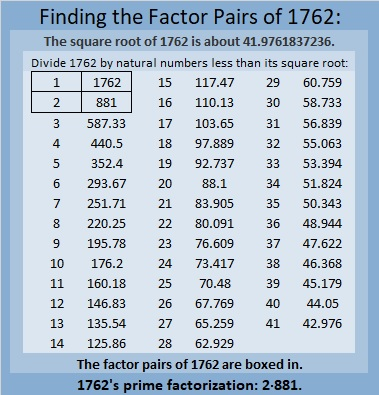### More About the Number 1762:

1762 is the sum of two squares:
41² + 9² = 1762.

1762 is the hypotenuse of a Pythagorean triple:
738-1600-1762 calculated from 2(41)(9), 41² – 9², 41² + 9².
It is also 2 times (369-800-881).

1762 is palindrome 7C7 in base 15
because 7(15²) + 12(15) + 7(1) = 1762.

# 1757 A Shillelagh Puzzle

### Today’s Puzzle:

When I was in elementary school, one of my teachers had us listen to a variety of songs for Saint Patrick’s Day including Bing Crosby’s It’s the Same Old Shillelagh. It’s almost Saint Patrick’s Day, so here’s a puzzle that looks a little like a shillelagh, an Irish walking stick.

Since this is a level-3 puzzle, begin with the clues at the top of the puzzle and work your way down using each number 1 to 12 once in the first column and once in the top row. The numbers you write and the given clues need to form a multiplication table. There is only one solution.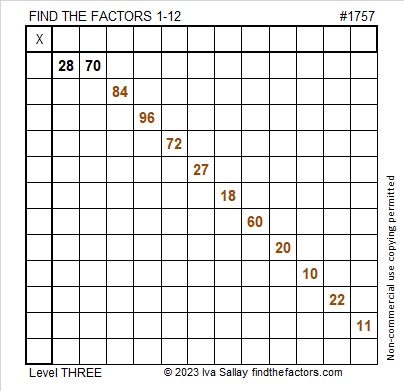Here is the same puzzle in black and white:### Factors of 1757:

• 1757 is a composite number.
• Prime factorization: 1757 = 7 × 251.
• 1757 has no exponents greater than 1 in its prime factorization, so √1757 cannot be simplified.
• The exponents in the prime factorization are 1 and 1. Adding one to each exponent and multiplying we get (1 + 1)(1 + 1) = 2 × 2 = 4. Therefore 1757 has exactly 4 factors.
• The factors of 1757 are outlined with their factor pair partners in the graphic below.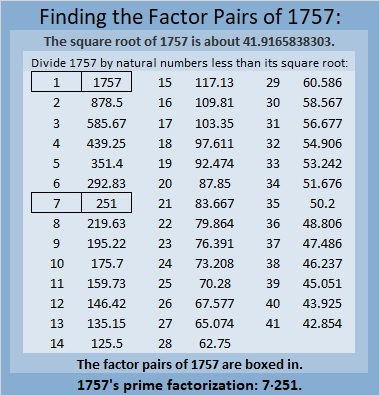### More About the Number 1757:

1757 is the difference of two squares in two different ways:
879² – 878² = 1757, and
129² – 122² = 1757.

1757 is palindrome 616 in base17
because 6(17²) + 1(17) + 6(1) = 1757.

# 1610 Four-Leaf Clovers

### Today’s Puzzle:

Sometimes four-leaf clovers are associated with Saint Patrick’s Day. Four-leaf clovers are supposed to be lucky, but you might not feel so lucky as you work on solving this puzzle. I assure you, there is a logical way to proceed on each step!Print the puzzles or type the solution in this excel file: 14 Factors 1604-1612.

### Factors of 1610:

• 1610 is a composite number.
• Prime factorization: 1610 = 2 × 5 × 7 × 23.
• 1610 has no exponents greater than 1 in its prime factorization, so √1610 cannot be simplified.
• The exponents in the prime factorization are 1, 1, 1, and 1. Adding one to each exponent and multiplying we get (1 + 1)(1 + 1)(1 + 1)(1 + 1) = 2 × 2 × 2 × 2 = 16. Therefore 1610 has exactly 16 factors.
• The factors of 1610 are outlined with their factor pair partners in the graphic below.### More about the Number 1610:

1610 is the hypotenuse of a Pythagorean triple:
966-1288-1610, which is (3-4-5) times 322.

1610 is not the sum of two squares or the difference of two squares. 1610 is a leg in some Pythagorean triples because
2(805)(1) = 1610,
2(161)(5) = 1610,
2(115)(7) = 1610, and
2(35)(23) = 1610.

You can calculate those Pythagorean triples by letting a be the first number in parenthesis for each of those equations, and b be the second number in parenthesis. Then substitute those values in the three expressions below, and you will have some Pythagorean triples!
2(a)(b), a² – b², a² + b².

# 1607 Shillelagh

### Today’s Puzzle:

A Shillelagh is an Irish wooden walking stick. This Shillelagh is keeping with our Saint Patrick’s Day theme, but it is a Find the Factors 1 to 14 puzzle.  Brutal! It will be a whole lot less tricky for you to solve because I made it a level 3 puzzle: The logic needed to solve the puzzle is built in. Just start with the clue at the top of the puzzle and work your way down cell by cell until you have found all the factors. So crack on!Print the puzzles or type the solution in this excel file: 14 Factors 1604-1612.

### Factors of 1607:

• 1607 is a prime number.
• Prime factorization: 1607 is prime.
• 1607 has no exponents greater than 1 in its prime factorization, so √1607 cannot be simplified.
• The exponent in the prime factorization is 1. Adding one to that exponent we get (1 + 1) = 2. Therefore 1607 has exactly 2 factors.
• The factors of 1607 are outlined with their factor pair partners in the graphic below.How do we know that 1607 is a prime number? If 1607 were not a prime number, then it would be divisible by at least one prime number less than or equal to √1607. Since 1607 cannot be divided evenly by 2, 3, 5, 7, 11, 13, 17, 19, 23, 29, 31, or 37, we know that 1607 is a prime number.

### More about the Number 1607:

1607 is the sum of two consecutive numbers:
803 + 804 = 1607.

1607 is also the difference of two consecutive numbers:
804² – 803² = 1607.

Did you notice what happened there? Try this next one:

1607² = 2582449.

1607²/2 = 1291224.5.

(1607-1291224-1291225) is a primitive Pythagorean triple.

Cool, isn’t it?

# 1458 Tangrams Can Be A Pot of Gold

### A Tangram Puzzle

Tangrams are seven puzzle pieces that can form a square but can also be made into many different people, places, and things. A lot of stress is going on in the world right now, but since tomorrow is Saint Patrick’s Day, we can still find a little pot of gold at the end of the rainbow!

I made this pot of gold on Desmos using points and equations. If you cut it apart, will you be able to put it back together again?What other things can you make from those seven tangram shapes?

And what about that rainbow I mentioned? The number 1458 makes a lovely factor rainbow.

#### A Factor Rainbow for 1458: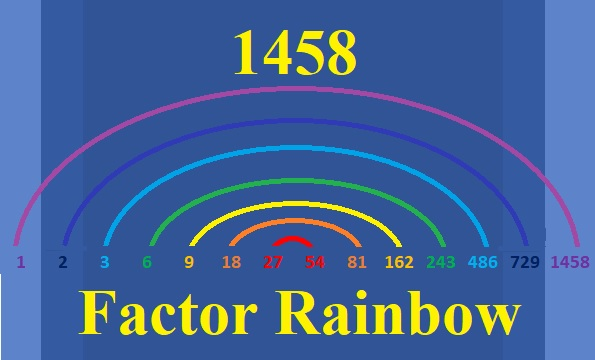#### Factors of the number 1458:

• 1458 is a composite number.
• Prime factorization: 1458 = 2 × 3 × 3 × 3 × 3 × 3 × 3, which can be written 1458 = 2 × 3⁶.
• 1458 has at least one exponent greater than 1 in its prime factorization so √1458 can be simplified. Taking the factor pair from the factor pair table below with the largest square number factor, we get √1458 = (√729)(√2) = 27√2
• The exponents in the prime factorization are 1 and 6. Adding one to each exponent and multiplying we get (1 + 1)(6 + 1) = 2 × 7 = 14. Therefore 1458 has exactly 14 factors.
• The factors of 1458 are outlined with their factor pair partners in the graphic below.#### More Facts about the Number 1458:

2 is a prime factor of 1458 exactly one time, so there are NO ways that 1458 can be written as the difference of two squares.

2 and 3 are the only primes appearing in its prime factorization, so 1458 is NEVER the hypotenuse of a Pythagorean triple.

Nevertheless, since there are three different ways that 1458 = 2(a)(b), where a > b, there are three ways that 1458 is a leg in a Pythagorean triple:
1458-531440-531442, calculated from 2(729)(1), 729² – 1², 729² + 1²
1458-59040-59058, calculated from 2(243)(3), 243² – 3², 243² + 3²
1458-6480-6642, calculated from 2(81)(9), 81² – 9², 81² + 9²

Why can’t we get a Pythagorean triple from 1458 = 2(27)(27)? I’m sure you can figure out that one yourself.

# 1036 Look, Look to the Rainbow

Finian’s Rainbow is a wonderful movie to enjoy on Saint Patrick’s Day. One of its songs reminds us to “Look, look to the rainbow”.

If you look to this rainbow, you will find all the factors of 1036:There is a simple symmetry in every rainbow. There is also symmetry in palindromes which are numbers, words, or sentences that read the same forward or backward.

1036 demonstrates that symmetry when it is written in some other bases:
It’s repdigit 4444 in BASE 6 because 4(6³ + 6² + 6¹ + 6⁰) = 4(216 + 36 + 6 + 1) = 4(259) = 1036,
It’s 232 in BASE 22 because 2(22²) + 3(22) + 2(1) = 1036,
1M1 in BASE 23 (M is 22 base 10) because 23² + 22(23) + 1 = 1036, and
SS in BASE 36 (S is 28 base 10) because 28(36 + 1) = 28(37) = 1036

1036 is also the hypotenuse of a Pythagorean triple:
336-980-1036 which is 28 times 12-35-37

• 1036 is a composite number.
• Prime factorization: 1036 = 2 × 2 × 7 × 37, which can be written 1036 = 2² × 7 × 37
• The exponents in the prime factorization are 2, 1, and 1. Adding one to each and multiplying we get (2 + 1)(1 + 1)(1 + 1) = 3 × 2 × 2 = 12. Therefore 1036 has exactly 12 factors.
• Factors of 1036: 1, 2, 4, 7, 14, 28, 37, 74, 148, 259, 518, 1036
• Factor pairs: 1036 = 1 × 1036, 2 × 518, 4 × 259, 7 × 148, 14 × 74, or 28 × 37,
• Taking the factor pair with the largest square number factor, we get √1036 = (√4)(√259) = 2√259 ≈ 32.18695There wasn’t a pot of gold at the end of our factor rainbow, but there is one here at the end of this post. It’s a level 5 puzzle, but it isn’t too difficult, so see if you can find all the factors that make the puzzle function like a multiplication table.Print the puzzles or type the solution in this excel file: 10-factors-1035-1043